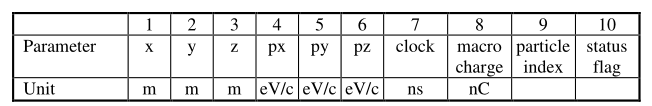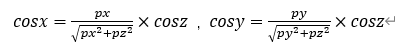# About the external phase space file

Dear FLUKA experts,
I have a question about how to calculate the starting X direction cosine of single particle in the topic of external phase space file. I want to import the particle information output by Astra into FLUKA via a phase space file.The format of the particle information output by Astra is as fig1.px, py, and pz are the momentum relative to the central particle.All three variables have positive and negative values.I now calculate the starting X, Y, Z directions cosine of single particle using Fig2.cosz=-1 when pz is negative, cosz=1 when pz is positive, calculate cosx, cosy.Due to the forward transmission towards the z-axis, all cosz in the phase space file are 1.May I ask if this calculation is correct？Best,
An

Dear An,

As far as I can understand, the first line of the ASTRA particle list file is the reference particle, and for any particle below it, the longitudinal parameters are relative to the reference particle.

For the reference particle the direction cosines are cos_x = cos_y = 0, cos_z = 1

For any subsequent particle, you need to calculate the absolute z-momentum and the total momentum first:

p_{z-abs} = p_z + p_{z-ref}
p = \sqrt{{p_x}^2 + {p_y}^2 + {p_{z-abs}}^2}

Then you can calculate the direction cosines simply:

cos_x = \frac{p_x}{p}, cos_y = \frac{p_y}{p}, cos_z = \frac{p_{z-abs}}{p}

Cheers,
David

Dear David,
Thank you so much for helping me solve a problem I’ve been thinking about for a long time.

Cheers,
An